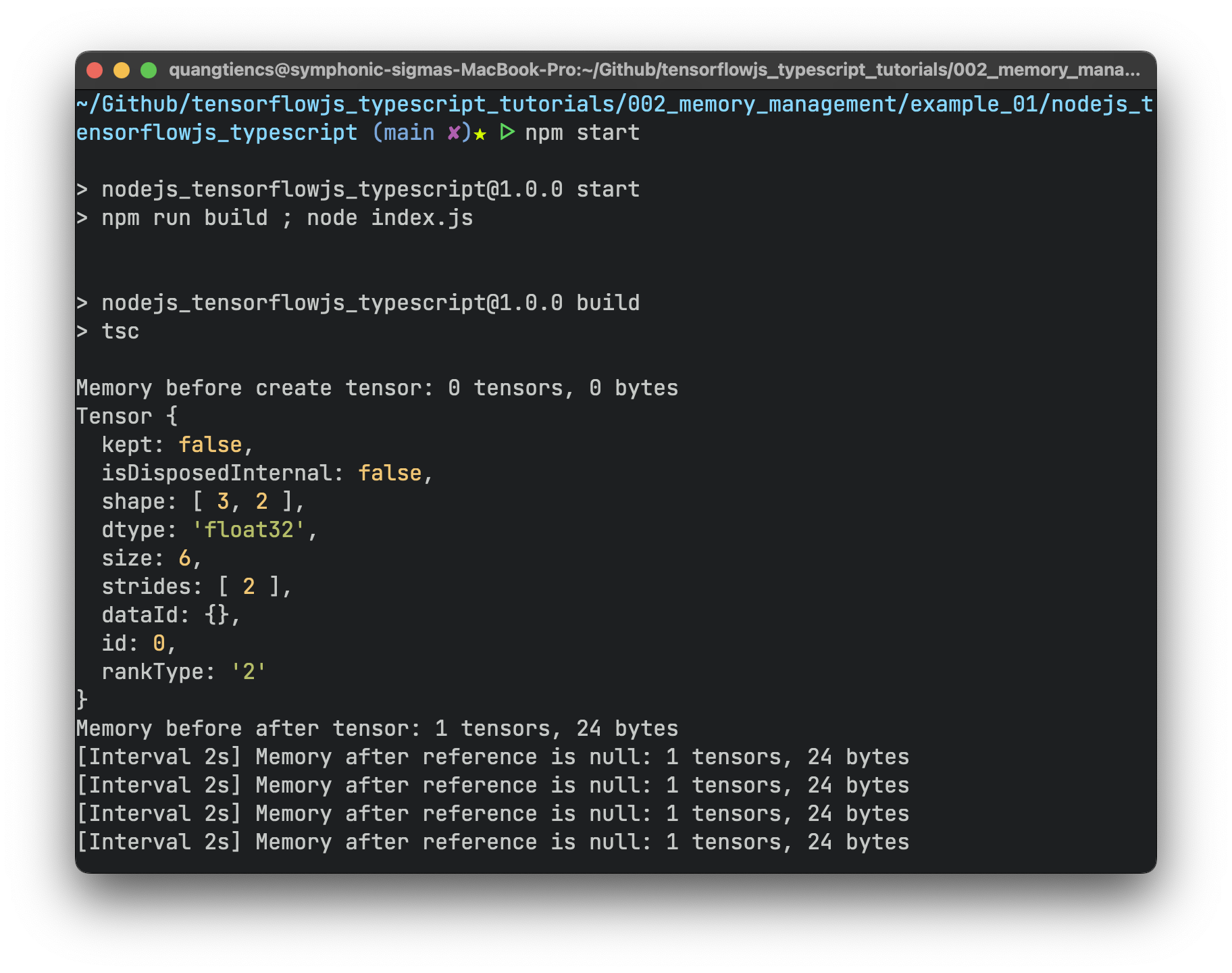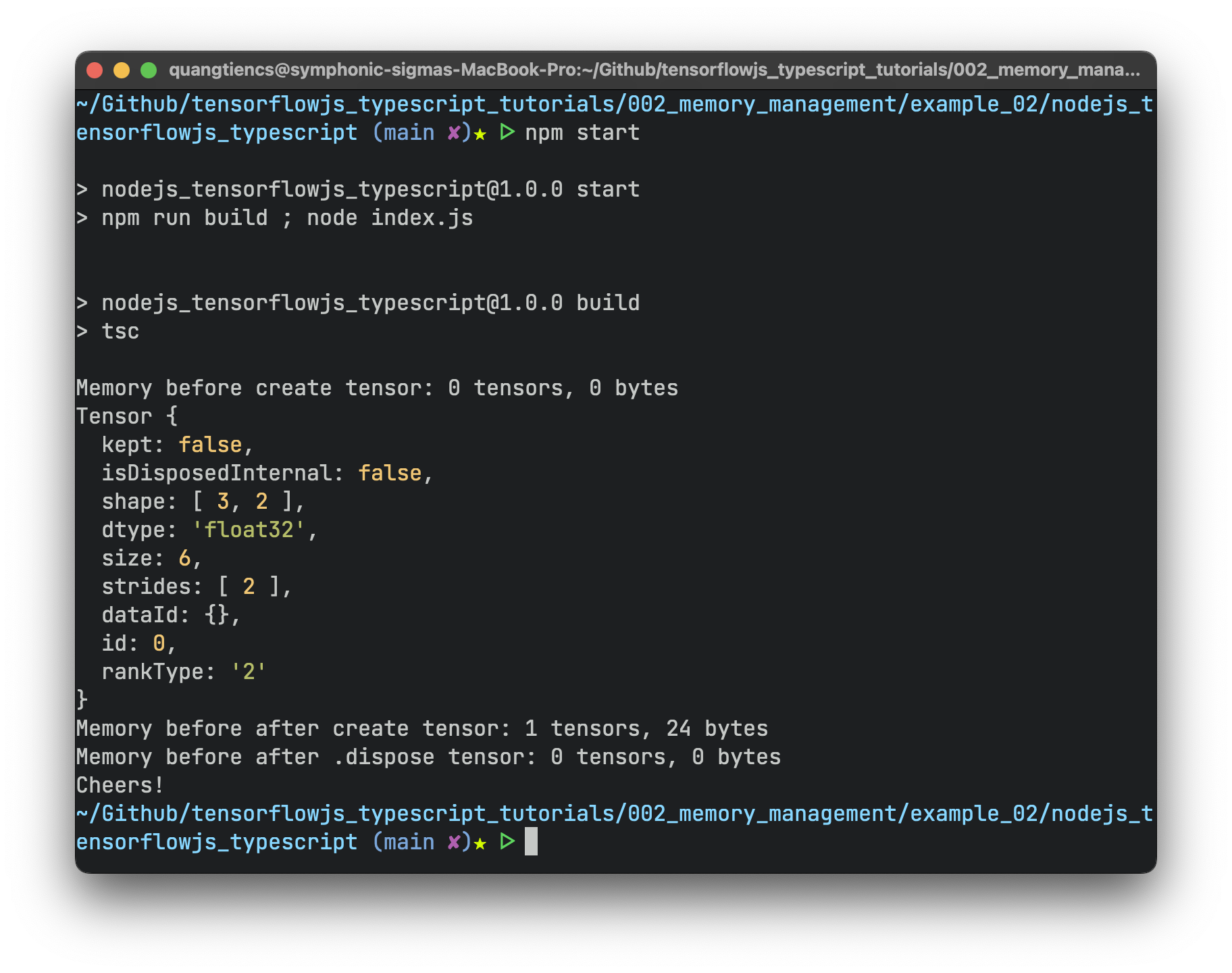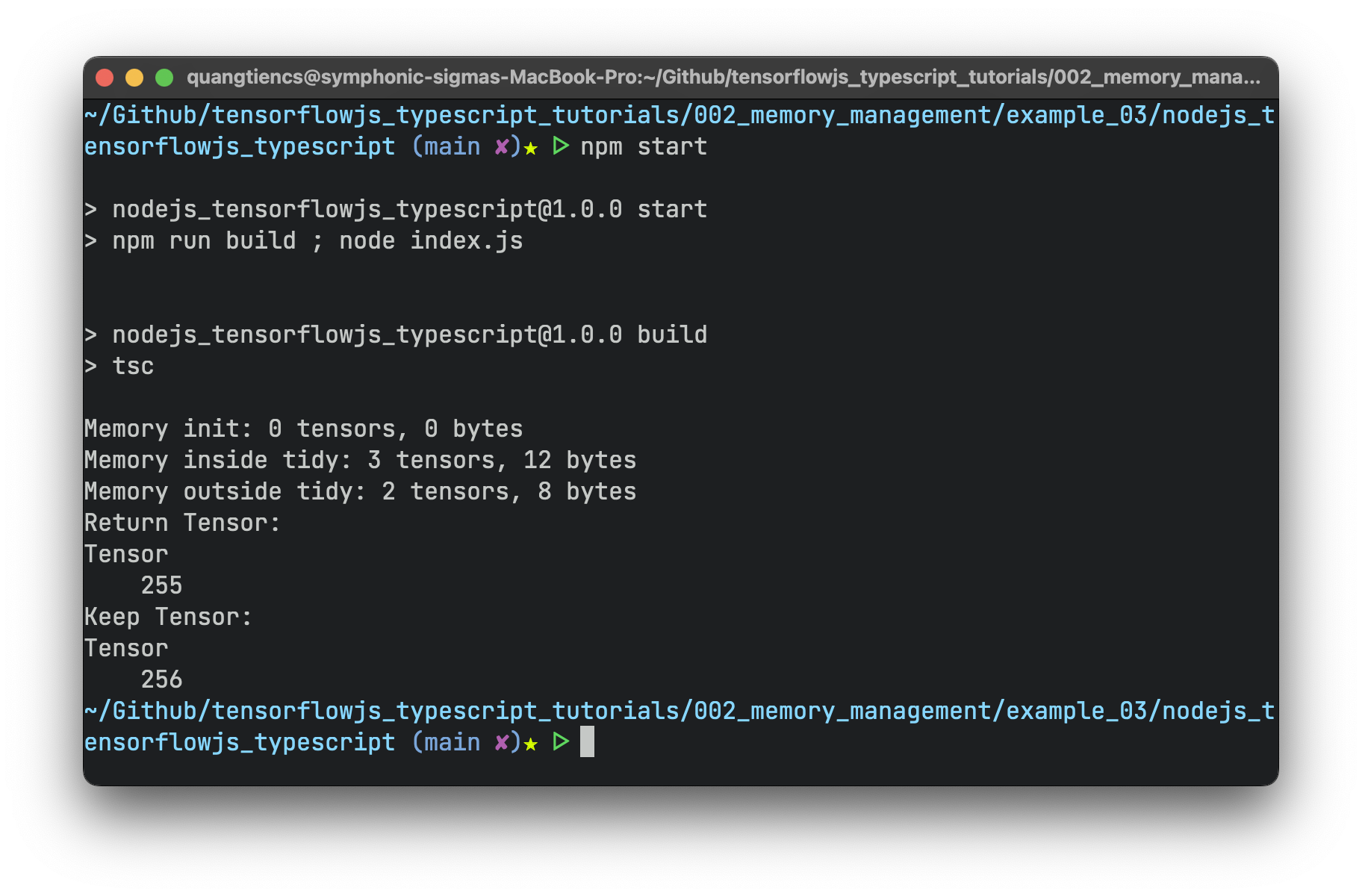Management Memory is essential for every program to work efficiently. Although Javascript has a Garbage Collector, our programs with TensorflowJS don’t get the same automatic memory management.

The tensor objects are persistent with the memory, although the javascript variable has no reference. This lead to memory leak problem.

Let’s understand the problem deeper through examples!

## 1. Memory information:

Sometimes you need to get your memory information, and these functions are helpful:

• `tf.memory().numTensors`: count the number of tensors we created.
• `tf.memory().numBytes`: count total bytes usage memory.

## 2. Allocating tensors & Deallocating tensors:

Do a small experiment 1:

``````import { Tensor, tensor2d, Tensor2D } from "@tensorflow/tfjs-node"
import * as tf from '@tensorflow/tfjs-node';

console.log(`Memory before create tensor: \${tf.memory().numTensors} tensors, \${tf.memory().numBytes} bytes`)
var t1: Tensor2D | null = tensor2d([[1, 2], [3, 4], [5, 6]])
console.log(t1)
console.log(`Memory before after tensor: \${tf.memory().numTensors} tensors, \${tf.memory().numBytes} bytes`)
t1 = null;

function intervalFunc() {
console.log(`[Interval 2s] Memory after reference is null: \${tf.memory().numTensors} tensors, \${tf.memory().numBytes} bytes`);
}

setInterval(intervalFunc, 2000);``````No matter how long you run, the usage memory is the same! We can manually clean up memory by using the helper function `.dispose()`.

Do a small experiment 2:

``````import { Tensor, tensor2d, Tensor2D } from "@tensorflow/tfjs-node"
import * as tf from '@tensorflow/tfjs-node';

console.log(`Memory before create tensor: \${tf.memory().numTensors} tensors, \${tf.memory().numBytes} bytes`)
var t1: Tensor2D | null = tensor2d([[1, 2], [3, 4], [5, 6]])
console.log(t1)
console.log(`Memory before after create tensor: \${tf.memory().numTensors} tensors, \${tf.memory().numBytes} bytes`)
t1.dispose();
console.log(`Memory before after .dispose tensor: \${tf.memory().numTensors} tensors, \${tf.memory().numBytes} bytes`)
console.log("Cheers!")``````There is another more convenient option! You can use `tf.dispose(containerVariable)` to deallocate all tensor variables. Read it:

## 3. Tidy scope:

TensorflowJS provides two helpful functions that make it easier to manage memory, as described in the documentation:

• tf.tidy tensorflow.js documentation : Executes the provided function `fn` and after it is executed, cleans up all intermediate tensors allocated by `fn` except those returned by `fn`. fn must not return a `Promise` (`async functions` not allowed). The returned result can be a complex object. Using this method helps avoid memory leaks.

• tf.keep tensorflow.js documentation : Keeps a `tf.Tensor` generated inside a `tf.tidy()` from being disposed automatically.

Do a small experiment 3:

``````import * as tf from '@tensorflow/tfjs-node';

console.log(`Memory init: \${tf.memory().numTensors} tensors, \${tf.memory().numBytes} bytes`)

var keepTensor: tf.Tensor | null = null;

const returnTensor = tf.tidy(() => {
const one = tf.scalar(1);
const a = tf.scalar(16);

keepTensor = tf.keep(a.square());

console.log(`Memory inside tidy: \${tf.memory().numTensors} tensors, \${tf.memory().numBytes} bytes`)

return keepTensor.sub(one);
});

console.log(`Memory outside tidy: \${tf.memory().numTensors} tensors, \${tf.memory().numBytes} bytes`)

console.log('Return Tensor:');
returnTensor.print();
console.log('Keep Tensor:');
keepTensor!!.print();``````